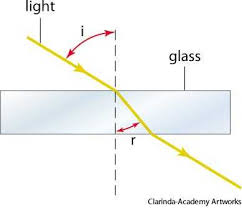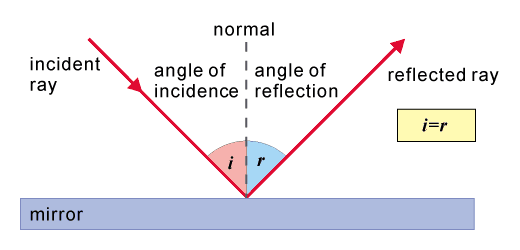# Changing Direction

## Refraction

All waves refract. Refraction occurs when a wave, let’s say light, travels through a medium (substance) of a different density. This causes light to either slow down (more dense) or speed up (less dense). This in turn makes the light change direction if it entered the medium at an angle.

In short, refraction occurs when light changes direction when travelling through a new medium of a different density.• The dotted line in the middle is called__ the normal__. This line is __perpendicular __to the surface of the substance and it meets the points where the wave enter or exits the substance.
• ‘i’ is the angle of incidence and is always measured between the ray and the normal
• ‘r’ is the __angle of refraction __and is always measured between the ray and the normal.

Notice how when light slows down it bends or refracts towards the normal and when it speeds up it bends or refracts away from the normal.

If light meets the boundary along the normal line (at 90 degrees) it does not bend and travels straight through.

## Reflection

All waves reflect. If the wave is a sound wave we call that an __echo. __The law of reflection is as follows:

__The angle of incidence = The angle of reflection __(as shown below in the diagram)

This law always holds.You may have to draw this out in an exam. Be sure to include arrows to show the direction that light is travelling.

What is the law of reflection?
Your answer should include: Angle of incidence equals the angle of reflection / Angle of incidence = angle of reflection
. Light travels into a denser medium, will it: a) Speed up and bend towards the normal, b) Speed up and bend away from the normal, c) Slow down and bend towards the normal, d) Slow down and bend away from the normal, e) None of the above
c
Light travels into a less dense medium at right angles, will it: a) Speed up and bend towards the normal, b) Speed up and bend away from the normal, c) Slow down and bend towards the normal, d) Slow down and bend away from the normal, e) None of the above
e